## 2.3.3 Short-time thermal description: stochastic LLG equations

From a general point of view, the dynamics of a system described by the variablein contact with a heat bath of temperature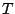are called Langevin dynamics(LD) and are described by the stochastic equations:(2.53)

whereis the noise term,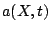the drift and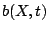the diffusion term.

In the magnetic systems it is customary to introduce thermal fluctuations as additional random field. The noise is supposed to be uncorrelated in time due to the fact that magnons are slower than phonons. It can be proven [Chubykalo 03b] that there are no correlations between components x,y,z and different particles. Therefore, the thermal fieldis a gaussian distributed noise with the following properties:(2.54)(2.55)

The fluctuation-dissipation theory, which states that the system response to a external excitation is equal to the response to a fluctuation, yields a value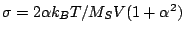[Brown 63b] that explicitly depends on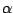.

The stochastic equation has to be numerically integrated. The general noise is represented by a function, which is continuous everywhere but not differentiable and its integralcan not be interpreted as a calculus integration. In the stochastic LLG equation this variable is the thermal field. The implementation of the integration gives rise to different calculi: the use of the value at the beginning of the step is known as Ito stochastic calculus and a midpoint integration as Stratonovich stochastic calculus and other implementations are also possible. Between the different schemes to integrate the stochastic equation, it has been argued that only the Heun scheme, which implies a midpoint integration and will be used here, gives the proper thermal properties [García-Palacios 98]. Posteriorly, D. Berkov [Berkov 02] demonstrated that in cartesian coordinates all the schemes are equivalent because a normalization is needed after each step in order to conserve the magnitude. E. Martinez et al. [Martínez 04] arrived to an equivalent conclusion in spherical coordinates. The Heun scheme is a predictor-corrector method. The first step or predictor is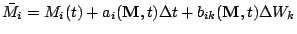(2.56)

and the second or corrector is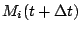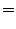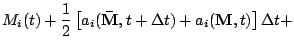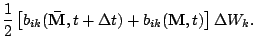(2.57)

The magnetization value will correspond in average to a minimum, but its actual position never rests in the minimum, the position that would reach without the thermal fluctuations. The numerical integration of the stochastic LLG equation does not allow, similar to its deterministic equivalent, simulation times larger than few nanoseconds and has also the inconvenience that prevents the use of advanced numerical schemes used in ordinary differential equations. Nevertheless, new schemes have been recently suggested [Martínez 07], which allow a longer timestep. The inclusion of the temperature in the micromagnetism is not straightforward and is focus of much interest. Namely, the micromagnetic integration of the stochastic LLG shows strong dependence on the discretization length [Tsiantos 02] and results in an overestimation of the Curie temperature [Grinstein 03].

2008-04-04